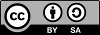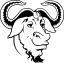# Note: these pages are no longer maintained

Never the less, much of the information is still relevant.
Beware, however, that some of the command syntax is from older versions, and thus may no longer work as expected.
Also: external links, from external sources, inside these pages may no longer function.Recipe #17 Railways vs Populated Places 2011 January 28

The problem

We'll use once again the railways dataset.
But this really is an hot spiced recipe: be prepared to taste very strong flavors.
As you can now easily image by yourself, computing distances between a railway line and Populated Places isn't so difficult.
So this problem introduces a further degree of complexity (just to escape from boredom and to keep your mind active and interested).

Image that for any good reason the following classification exists:
 Class Min. distance Max. distance A-class 0 Km 1 Km B-class 1 Km 2.5 Km C-class 2.5 Km 5 Km D-class 5 Km 10 Km E-class 10 Km 20 Km
The problem you are faced to resolve is:
• identify any Populated Place laying within a distance radius of 20 Km from a Railway.
• identify the corresponding distance Class for each one of such Populated Places.

 Railway PopulatedPlace A class [< 1Km] B class [< 2.5Km] C class [< 5Km] D class [< 10Km] E class [< 20Km] Ferrovia Adriatica Zapponeta NULL NULL NULL NULL 1 Ferrovia Adriatica Villamagna NULL NULL NULL NULL 1 Ferrovia Adriatica Villalfonsina NULL NULL NULL 1 0 Ferrovia Adriatica Vasto 1 0 0 0 0 ... ... ... ... ... ... ...

 SELECT rw.name AS Railway,   pp_e.name AS PopulatedPlace,   (ST_Distance(rw.geometry,     Transform(pp_a.geometry, 23032)) <= 1000.0)       AS "A class [< 1Km]",   (ST_Distance(rw.geometry,     Transform(pp_b.geometry, 23032)) > 1000.0)       AS "B class [< 2.5Km]",   (ST_Distance(rw.geometry,     Transform(pp_c.geometry, 23032)) > 2500.0)       AS "C class [< 5Km]",   (ST_Distance(rw.geometry,     Transform(pp_d.geometry, 23032)) > 5000.0)       AS "D class [< 10Km]",   (ST_Distance(rw.geometry,     Transform(pp_e.geometry, 23032)) > 10000.0)       AS "E class [< 20Km]" FROM railways AS rw JOIN populated_places AS pp_e ON (   ST_Distance(rw.geometry,     Transform(pp_e.geometry, 23032)) <= 20000.0   AND pp_e.id IN (     SELECT pkid     FROM idx_populated_places_geometry     WHERE pkid MATCH RTreeIntersects(       MbrMinX(         Transform(           ST_Envelope(rw.geometry), 4326)),       MbrMinY(         Transform(           ST_Envelope(rw.geometry), 4326)),       MbrMaxX(         Transform(           ST_Envelope(rw.geometry), 4236)),       MbrMaxY(         Transform(           ST_Envelope(rw.geometry), 4326))))) LEFT JOIN populated_places AS pp_d ON (   pp_e.id = pp_d.id   AND ST_Distance(rw.geometry,     Transform(pp_d.geometry, 23032)) <= 10000.0   AND pp_d.id IN (     SELECT pkid     FROM idx_populated_places_geometry     WHERE pkid MATCH RTreeIntersects(       MbrMinX(         Transform(           ST_Envelope(rw.geometry), 4326)),       MbrMinY(         Transform(           ST_Envelope(rw.geometry), 4326)),       MbrMaxX(         Transform(           ST_Envelope(rw.geometry), 4236)),       MbrMaxY(         Transform(           ST_Envelope(rw.geometry), 4326))))) LEFT JOIN populated_places AS pp_c ON (   pp_d.id = pp_c.id   AND ST_Distance(rw.geometry,     Transform(pp_c.geometry, 23032)) <= 5000.0   AND pp_c.id IN (     SELECT pkid     FROM idx_populated_places_geometry     WHERE pkid MATCH RTreeIntersects(       MbrMinX(         Transform(           ST_Envelope(rw.geometry), 4326)),       MbrMinY(         Transform(           ST_Envelope(rw.geometry), 4326)),       MbrMaxX(         Transform(           ST_Envelope(rw.geometry), 4236)),       MbrMaxY(         Transform(           ST_Envelope(rw.geometry), 4326))))) LEFT JOIN populated_places AS pp_b ON (   pp_c.id = pp_b.id   AND ST_Distance(rw.geometry,     Transform(pp_b.geometry, 23032)) <= 2500.0   AND pp_b.id IN (     SELECT pkid     FROM idx_populated_places_geometry     WHERE pkid MATCH RTreeIntersects(       MbrMinX(         Transform(           ST_Envelope(rw.geometry), 4326)),       MbrMinY(         Transform(           ST_Envelope(rw.geometry), 4326)),       MbrMaxX(         Transform(           ST_Envelope(rw.geometry), 4236)),       MbrMaxY(         Transform(           ST_Envelope(rw.geometry), 4326))))) LEFT JOIN populated_places AS pp_a ON (   pp_b.id = pp_a.id   AND ST_Distance(rw.geometry,     Transform(pp_a.geometry, 23032)) <= 1000.0   AND pp_a.id IN (     SELECT pkid     FROM idx_populated_places_geometry     WHERE pkid MATCH RTreeIntersects(       MbrMinX(         Transform(           ST_Envelope(rw.geometry), 4326)),       MbrMinY(         Transform(           ST_Envelope(rw.geometry), 4326)),       MbrMaxX(         Transform(           ST_Envelope(rw.geometry), 4236)),       MbrMaxY(         Transform(           ST_Envelope(rw.geometry), 4326)))));
Yes, this one really looks like a complex and intimidating query.
Anyway, complexity is much more apparent than real.
You already know the trick: you simply have to break down this statement into several smallest chunks. And then you'll soon discover that there isn't nothing really difficult and complex.

Let us examine the main framework:
 SELECT rw.name AS Railway, ... FROM railways AS rw JOIN populated_places AS pp_e ON (...) LEFT JOIN populated_places AS pp_d ON (...) LEFT JOIN populated_places AS pp_c ON (...) LEFT JOIN populated_places AS pp_b ON (...) LEFT JOIN populated_places AS pp_a ON (...);
• we'll simply JOIN the railways AS rw and the populated_places AS pp_e tables: and there is nothing strange in this, isn't ?
• then we'll LEFT JOIN the populated_places AS pp_d table a second time: and you surely remember that LEFT JOIN inserts a valid row into the result-set even when the right-sided term evaluates to NULL.
• and finally we'll repeat LEFT JOIN for pp_c, pp_b and pp_a.
• we'll obviously start by checking the biggest distance, because if this one fails any other comparison will surely fail as well.
And so on, following the decreasing distance sequence.
 ... JOIN populated_places AS pp_e ON (   ST_Distance(rw.geometry,     Transform(pp_e.geometry, 23032)) <= 20000.0 ...
• each JOIN (or LEFT JOIN) simply evaluates the distance intercurring between the Railway line and the Populated Place, checking if this one falls within the expected threshold for the corresponding Class.
• using Transform() is required because the railway is in the 23032 SRID, whilst the populated place is in the 4326 SRID.
 ... AND pp_e.id IN (   SELECT pkid   FROM idx_populated_places_geometry   WHERE pkid MATCH RTreeIntersects(     MbrMinX(       Transform(         ST_Envelope(rw.geometry), 4326)),     MbrMinY(       Transform(         ST_Envelope(rw.geometry), 4326)),     MbrMaxX(       Transform(         ST_Envelope(rw.geometry), 4236)),     MbrMaxY(       Transform(         ST_Envelope(rw.geometry), 4326))) ...
• using the usual Spatial Index handling stuff is required anyway, so to support fast filtering of Populated Places geometries.
• and obviously we have to apply Transform(), so to reproject the railway geometry into the 4326 SRID (the one used by Populated Places).
• Please note well: applying a coordinate's transformation to a single POINT can be considered a light-weight op;
but transforming (many and many times …) some complex LINESTRING or POLYGON is much more an heavy-weight op.
So we'll smartly use ST_Envelope() in order to strongly simplify any geometry requiring transformation.
 All right, now the main framework of the complex query is absolutely clear: the first JOIN will include into the result-set any Populated Place falling within 20 Km from the railway line. any other LEFT JOIN will then test decreasing distances, accordingly to the imposed Class boundaries. and each LEFT JOIN carefully checks if the Populated Place ID is the same of the previous successfully identified Class, as in: pp_d.id = pp_c.id each time one such LEFT JOIN will fail, then corresponding NULL-values will be inserted into the result-set.

 SELECT rw.name AS Railway,   pp_e.name AS PopulatedPlace,   (ST_Distance(rw.geometry,     Transform(pp_a.geometry, 23032)) <= 1000.0)       AS "A class [< 1Km]", ...
Just a latest element to be shortly explained:
• consider a distance of e.g. 3.8 Km: this requires inclusion in C-class. But this condition satisfies LEFT JOIN matching criteria for D-class and E-class as well.
• we have obviously to perform a further check.
• happily SQL is a really smart language. Any selected column to be returned into the result-set may represent any appropriate arbitrary expression.
• and in SQL a logical expression evaluates as:
• 0 [FALSE]
• 1 [TRUE]
• or NULL, if anyone of the evaluated operands was NULL.
• and that's really all.
 You can now play by yourself, performing further tests on this query. i.e you can add some smart ORDER BY or WHERE clause and so on: that's really easy now, isn't ?Author: Alessandro Furieri a.furieri@lqt.it This work is licensed under the Attribution-ShareAlike 3.0 Unported (CC BY-SA 3.0) license.Permission is granted to copy, distribute and/or modify this document under the terms of the GNU Free Documentation License, Version 1.3 or any later version published by the Free Software Foundation; with no Invariant Sections, no Front-Cover Texts, and no Back-Cover Texts.